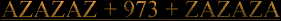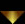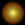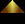Fingerprints of the gods Page280 To Re cap said Zed Aliz Yuga               =   12000 divine years x 360   =   4320000         Krita Yuga      =     4800 divine years x 360   =    1728000 Treta Yuga      =     3600 divine years x 360   =     1296000 Davpara Yuga =     2400 divine years x 360   =       864000 Kali Yuga        =     1200 divine years x 360   =       432000 432000 x Mahayuga = 3456000 ÷ 360 = 9600                                       3456000 ÷   72 = 48000                                       3456000 ÷   54 = 64000                                       3456000 ÷   45 = 76800                                       3456000 ÷  Ra and the eight = 192000                                       3456000 ÷  12  = 288000                                       3456000 ÷    9  = 384000                                       3456000 ÷    3  =  1152000                                                               432000 ÷ Mahayuga              = 54000 Krita x Yuga =20                   Krita x Yuga  x Treta x Yuga = 400 Krita x Yuga  x Treta x Yuga x Davpara x Yuga = 11200 Krita x Yuga  x Treta x Yuga x Davpara x Yuga x Kali x Yuga =179200 Krita x Treta x Davpara x Kali Krita x Treta = 25       Krita  x Treta x Davpara = 175 Krita  x Treta x Davpara x Kali =700 The very very very magic number seven scribe said ZedAliz The scribe simply writ seven as 7 Reight scribe said Alizzed Now add to deduce . The scribe writ reduce to adduce. Azin divine years. 1728000  = 18   = 9 1296000  = 18   = 9 864000    = 18   = 9 432000              = 9                              9 + 9 + 9 + 9    =    36  =  9                              9 x 9 x 9 x 9    =  656  =  17  = 8                                                                              8 x 9  = 72 Azin 9 said Zed aliz There is no logic to this Alizzed said the scribe Exactly scribe said Zed aliz still intently Looking for Kings. Yuga = 12000 divine years                      1 + 2   = 3 Krita Yuga = 4800 divine years  4 + 8 = 1 + 2   = 3 Treta Yuga  = 3600 divine years             3 + 6   = 9 Davpara Yuga = 2400 divine years         2 + 4   = 6 Kali Yuga = 1200 divine years               1 + 2   = 3                                                                              3  +  3 +  9 +  6 + 3 =  24  = 6                                       3  x  3  x 9  x 6  x 3 =   1458   = 18  = 9                                                                                     9  x  9                       9 x 6 = 54                                                                                         81 x 6                                                                                      486 x 3                       1458   Thus writ the scribe    Fingerprints of the Gods Page 280 KritaYuga        TretaYuga         DavparaYuga         KaliYuga    5  +  4               5  +  4                    7    +   4              4  +  4        9          x           9              x             11          x            8              9 x 9       81 x 11            891 x 8               7128 divided by Mahayuga is 891 Azin 8 x 9 x 1 is 72 Enough is not enough scribe said Zed AlizZed  time to move on The Puranas also tell us that 'one year of the mortals is equal to one day of the gods.'  Furthermore, and exactly as in the Osiris myth, we discover that the number of days in the years of both gods and mortals has been artificially set at 360, so one year of the gods is equivalent to 360 mortal years. Times                                                       360                            Times                           360 129600 divided  by  eighteen  is 72Fingerprints Of The Gods Page 273 These he joined to the 360 days of which the year then consisted (emphasis added). Elsewhere the myth informs us that the 360 -day year  consists of "12 months of 30 days each". Note 6 And in general,as Sellers observes , "phrases are used which prompt simple mental calculations and an attention to numbers ". note 7 Reight scribe said Zed Aliz write this down The scribe then writ as follows Osiris x Isis  is  24  Azin  6 Osiris x Isis x Set is 72 Azin 9 Osiris x Isis x Set x The other  is 360 Azin 9 Oh sir so it is  cried  the scribe,  said not to ZedAlizzed, and said  to nobody in particular . Page 280 The Kali Yuga, therefore, at 1200 years of the gods, turns out to have a duration of 432,000 mortal years.  One Mahayuga, or Great Age (made up of the 12,000 divine years contained in the four lesser Yugas) is equivalent to 4,320,000 years of mortals.  A thousand such Mahayugas (which constitute a Kalpa, or Day of Brahma) extend over 4,320,000,000 ordinary years, again supplying the digits for basic precessional calculations.  Separately there are Manvantaras (periods of Manu) of which we are told in the scriptures that 'about 71 systems of four Yugas elapse during each Manvantara.  The reader will recall that one degree of precessional motion along the ecliptic requires 71.6 years to complete, a number that can be rounded down to 'about 71' in India just as easily as it was rounded up to 72 in Ancient Egypt.     72 x Yugas = 360               432 ÷ 12      Mahayugas x Kalpa           Mahayugas x Brahma      Day of Brahma                  36                                  45                                        54        3 x 2  x  6          6  x 6            36 Brahma x Yuga x Kalpa = 120 Mahayugas x Brahma = 360 Manvantara  x 72 = 720 The scribe then out of nowhere , not suddenly, writ 71 x 71 is 5041 Page 281 "The Kali Yuga, with a duration of 432,000 mortal years, is, by the way, our own .  'In the Kali Age,' the scriptures say, 'shall decay flourish, until the human race approaches annihilation." 432000 ÷ Kali Yuga = 54000 'In the Kali Age,' 2  x  3  x  4  x  3      6  x  4         24 x 3             72 "In that land, as we have seen, Orion is Osiris, the god of death and resurrection, whose numbers - perhaps by chance - are 12, 30, 72, and 360." 12 x 30 x 72 x 360     360 x 72          25920 x 360                9331200                9331200 ÷  432  is  21600                9331200 ÷    12  is  777600                9331200 ÷    18  is  518400 12 + 30 + 72 + 360         is 474 Reight scribe said AlizZed  remove 474 from 504  and what is left The scribe said ,sixty six is left, so what. ?Ask a solemn Solomon said ZedAlizzed it's a revelation Osiris, the god of death and resurrection, whose numbers -- are 12, 30, 72, and 360." Add to reduce and thus deduce, sang a sing song ZedAlizZed. 1+2   3+0  7+2      3+6+0    3      3        9           9         3  x  3             9  x 9                81 x 9                   729÷18                      405 Chapter 33 Page 293      2 x 9 x 3                         3 x 3        1 x 9 + 9  x 3          18 x 3                            9                 9 + 9               54                                                  18 x 3                                                                         54 "Giza Egypt 16 March1993    3-30 pm" Just keep luck, look, looking, for coincidence, scribe, said Alizzed.  Snap Alizzed said the scribe, writing pans. Chapter 35 Page 310 Tombs and Tombs only ?   "Khufu,  Khafre,  Menkaure… Cheops,  Chephren,  Mycerinus. Whether they were referred to by their Egyptian or their Greek names, the fact remained that these three pharaohs of the Fourth Dynasty (2575 - 2467 BC ) were universally acclaimed as the builders of the Giza pyramids." The number of letters in Pharaohs name Khufu  Khafre  Menkaure                      Cheops    Chephren   Mycerinus      5            6               8                                    6                 8                 9      5  x  6 x  8 = 240                                          6  x  8 x 9            30 x 8                                                          48 x 9              240                                                              432                                            240 + 432                                                 672                                             6 + 7 + 2                                                  15                                                                                            1 + 5                                                   6        5 + 6 + 8 = 19                                              6 + 8 + 9 = 23                                              19 + 23                                                                                      42                                                4 + 2                                                   6 Khufu  x  Khafre  = 30                             48 =  Cheops  x  Chephren   Menkaure  x  Khufu = 40                      54  =   Mycerinus  x  Cheops   Khafre x  Menkaure   =  48                 72  =   Chephren  x Mycerinus 30 + 40 + 48                                                            48 + 54 + 72      70 + 48                                                                     54 + 72         118                                                                            126     1 + 1 + 8                                                                    1 + 2 + 6          10                                    x                                          9                                                 90   Khufu  x  Khafre  = 30                             48 =   Cheops  x  Chephren   Menkaure  x  Khufu = 40                        54  =  Mycerinus  x  Cheops   Khafre x  Menkaure   =  48                  72  =   Chephren  x Mycerinus Khufu  x  Khafre       = 30         +            48 =  Cheops  x  Chephren                                      3 + 0                   4 + 8                                    3             x            12                                                  36   Menkaure  x  Khufu = 40                        54  =   Mycerinus  x  Cheops                                      4 + 0                   5 + 4                                      4           x            9                                                  36    Khafre x  Menkaure    =  48                       72  =   Chephren  x Mycerinus                                    4 + 8                   7 + 2                                      12         x              9                                                108     "Khufu,  Khafre,  Menkaure… Cheops,  Chephren,  Mycerinus. Whether they were referred to by their Egyptian or their Greek names, the fact remained that these three pharaohs of the Fourth Dynasty (2575 - 2467 BC ) were universally acclaimed as the builders of the Giza pyramids." three pharaohs of the Fourth Dynasty (2575 - 2467 BC ) 2575 + 2467            2575 - 2467        5042                          108     5 + 4 + 2                      1+ 8            11              x              9                           99                                         9 x 9                           81                        8 + 1   three pharaohs of the Fourth Dynasty (2575                                     2467 BC )   2575 x  360                 360 x 2467       927000                          888120    9 + 2 + 7                     8 + 8 + 8 + 1 + 2         18                                      27       1 + 8                                  2 + 7          9                    +                   9                             is 18  Azin Ra and the illustrious company of gods.                             So said Alizzed .Thus writ the far yonder scribe. Page 310 cont… "Ancient Egyptian tour guides had told the Greek historian Herodotus that the Great Pyramid had been built by Khufu. Herodotus had incorporated this information into the oldest surviving written description of the monuments, which continued: Cheops , they said, reigned for fifty years, and on his death the kingship was taken over by his brother Chephren. He also made a pyramid… it is forty feet lower than his brother's pyramid, but otherwise of the same greatness… Chephren reigned for fifty-six years… then there succeeded Mycerinus, the son of Cheops… This man left a pyramid much smaller than his father's "       " Cheops , they said, reigned for " fifty years, 50 x 360 = 1800   "…it is forty feet lower than his brother's pyramid " 40 x 12 = 480   " Chephren reigned for fifty-six years " 56 x 360 = 20160   Acatalectic Acatalepsy Acateleptic